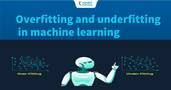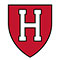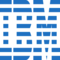Support Vector Machines: Python code

# Support Vector Machines: Python code

clickHere
Updated on Sep 26, 2022 10:15 IST

A support vector machine (SVM) is a supervised machine learning model that uses classification techniques for solving two-group classification problems. In this article, we will learn more about SVM using python codes.

Most newcomers start with regression and classification methods for machine learning. These algorithms are simple. However, understanding the fundamentals of machine learning requires going beyond these two machine learning algorithms. Machine learning has a lot more to offer than regression and classification, and it can assist us in solving a variety of complicated problems. Let us look at the Support Vector Machine Algorithm, one such algorithm. The Support Vector Machines algorithm, or SVM algorithm, is a machine learning technique that can solve both regression and classification problems with efficiency and accuracy.

## What are Support Vector Machines(SVM)?

It’s crucial to know the complex history of the SVM algorithm before learning how it solves classification and regression-based problems. Vladimir Vapnik created SVM in the 1970s. According to mythology, it was created as part of a wager in which Vapnik believed that devising a decision boundary that tries to maximize the margin between the two classes would yield excellent results and solve the overfitting problem.

A support vector machine (SVM) is a supervised machine learning model that uses classification techniques for solving two-group classification problems. SVM models can categorize new text after being given labeled training data sets for each category.

These models offer two key advantages over newer algorithms like neural networks: faster processing and better performance with fewer samples (in the thousands). Because of these features, SVM models are particularly well suited to deal with text classification issues, where you have access to a few thousand tagged samples.

Must Check: What is Machine Learning?Random Forest Algorithm: Python Code
This blog will explain you about random forest in machine learning with bagging and boosting techniquesOverfitting and Underfitting with a real-life exampleCross-validation techniques

## Key Terminologies of SVM

### 1. Hyperplane:

Hyperplanes, also known as decision boundaries or decision planes, are the boundaries that aid in the classification of data points. The side of the hyperplane where a new data point lands can be divided into multiple groups. The hyperplane’s dimension is determined by the number of features assigned to a dataset. The hyperplane can be a simple line if the dataset only has two features. A hyperplane is a two-dimensional plane when a dataset comprises three features.

### 2. Support Vectors:

Support vectors are the data points closest to the hyperplane and affect its position. These vectors are called support vectors because they alter hyperplane location, hence the Support Vector Machine Algorithm.

### 3. Margin:

The distance between the hyperplane and the support vectors is the margin. The hyperplane that optimizes the margin is always chosen using SVM. The wider the margin, the more accurate the results are. There are two types of margins in SVM algorithms: hard and soft.

SVM can select two parallel lines that maximize the marginal distance when the training dataset is linearly separable; this is known as a hard margin. The SVM admits some margin violations when the training dataset is not linearly separable. A soft margin permits some data points to remain on the wrong side of the hyperplane or between the margin and the hyperplane while maintaining accuracy.

## Types of SVM

### 1. Linear SVM:

For a linearly separable dataset, linear SVM is employed. A simple real-world example can help us comprehend how a linear SVM works. CFor a linearly separable dataset, linear SVM is employed. A simple real-world example can help us comprehend how a linear SVM works. Consider a dataset with only one feature: a person’s weight. Obese and non-obese data points are expected to be categorized into two categories. SVM may generate a maximal-margin hyperplane to organize data points into these two groups using the nearest support vectors. The SVM will now identify the hyperplane’s side, where it falls, and classify the person as fat or not every time a new data point is provided.

### 2. Non-linear SVM:

Separating the dataset linearly becomes difficult as the number of features grows. A non-linear SVM is used in this case. When the dataset is not linearly separable, we can’t draw a straight line to separate data points. SVM offers another dimension to distinguish these data points. Z = x2 + Y2 is the new dimension that can be determined. This calculation will aid in linearizing a dataset’s features, allowing SVM to generate a hyperplane to categorize data points.

When a data point is turned into a high-dimensional space by adding a new dimension, a hyperplane can readily separate it. This is accomplished via a technique known as the kernel trick. SVM algorithms can convert non-separable data into separable data using the kernel method.

## Demo on SVM

Here’s a small demo demonstrating the Support Vector Machines Algorithm. As a first step, import the necessary libraries. The data set used is breast_cancer_data.

` `
`#Load the necessary python librariesimport numpy as npimport pandas as pdimport matplotlib.pyplot as pltimport seaborn as snsfrom sklearn.model_selection import train_test_splitfrom sklearn.preprocessing import LabelEncoderfrom sklearn.preprocessing import MinMaxScalerfrom sklearn.svm import SVCfrom sklearn.metrics import accuracy_scoreCopy code`

The next step is to load the data and read the data from the file.

` `
`data = pd.read_csv('/content/Breast_cancer_data.csv')data.head()Copy code`

Now, let’s check for null values, if any.

` `
`data.isna().sum()Copy code`

Describing the data using describe() function.

` `
`data.describe()Copy code`

Checking out the information of the data

` `
`data.info()Copy code`

We are finding out the correlation between the features using heatmap.

` `
`corr = data.corr()fig = plt.figure(figsize=(15,12))a = sns.heatmap(corr, cmap='Oranges')a.set_title("Data Correlation")Copy code`

We define the dependent and independent variables and split them into training and testing sets as 70:30 ratio, respectively.

` `
`y = data["diagnosis"].valuesX=data.drop(["diagnosis"],axis=1)from sklearn.model_selection import train_test_splitX_train, X_test, y_train, y_test = train_test_split(X, y,test_size = 0.3,random_state=1)Copy code`

### Some important hyperparameters that should be considered before training the model:

1. C: The regularization parameter has a float value and is 1.0 by default. Must be strictly positive.
2. kernel: Specifies the kernel type used in the algorithm. ‘linear,’ ‘poly,’ ‘rbf.’
3. degree: An optional integer value to specify the degree of polynomial kernel function which is ignored by all other kernels
4. gamma: Kernel coefficient for ‘rbf’,’poly’
5. coef0: Independent term in kernel function significant in ‘poly.’
` `
`svc_diag = SVC(C=10,kernel='linear')Copy code`

We are checking the accuracy of the model.

` `
`predicted=svc_diag.predict(X_test)acc_svc=accuracy_score(y_test,predicted)print('Accuracy Score of Linear Model: ',acc_svc)Copy code`
` `
`svc_diag=SVC(C=10,kernel='rbf',gamma=2)svc_diag.fit(X_train,y_train)Copy code`

We are checking the accuracy of the model.

` `
`predicted=svc_diag.predict(X_test)acc_svc=accuracy_score(y_test,predicted)print('Accuracy Score of Gaussian Model: ',acc_svc)Copy code`

## Conclusion

This article looked at the Support Vector Machine Algorithm in depth. We learned about the SVM algorithm, its working, different types of SVM algorithms, and Python implementation.

clickHere

This is a collection of insightful articles from domain experts in the fields of Cloud Computing, DevOps, AWS, Data Science, Machine Learning, AI, and Natural Language Processing. The range of topics caters to upski... Read Full Bio

## Trending Data Science CoursesMachine Learning
Coursera4.6AI for Medicine Specialization
Coursera4.0Starts today

## Top Picks & New Arrivals